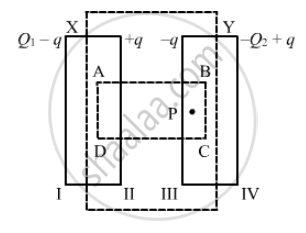Advertisement Remove all ads

# P Suppose a Charge +Q1 is Given to the Positive Plate and a Charge −Q2 to the Negative Plate of a Capacitor. What is the "Charge on the Capacitor"? - Physics

Short Note

Suppose a charge +Q1 is given to the positive plate and a charge −Q2 to the negative plate of a capacitor. What is the "charge on the capacitor"?

Advertisement Remove all ads

#### SolutionGiven :

Charge on the positive plane = +Q_1

Charge on the negative plate = -Q_2

To calculate: Charge on the capacitor

Let ABCD be the Gaussian surface such that faces AD and BC lie inside plates X and Y, respectively.
Let q be the charge appearing on surface II. Then, the distribution of the charges on faces I, III and IV will be in accordance with the figure.

Let the area of the plates be A and the permittivity of the free space be ∈_0.

Now, to determine q​ in terms of Q1 and Q2, we need to apply Gauss's law to calculate the electric field due to all four faces of the capacitor at point P. Also, we know that the electric field inside a capacitor is zero.

Electric field due to face I at point P, E1 = (Q_1 - q)/(2∈_0A)

Electric field due to face II at point P, E2= (+q)/(2∈_0A)

Electric field due to face III at point P, E3 = (-q)/{2∈_0A)

Electric field due to face IV at point P, E4 = -((-Q_2+q)/(2∈_0A))      (Negative sign is used as point P lies on the LHS of face IV.)

Since point P lies inside the conductor,

E1 + E2 + E3 + E4 = 0

therefore Q_1-q+q-q-(-Q_2+q) = 0

⇒ q = (Q_1+Q_2)/2

Thus , the change on the capacitor is (Q_1+Q_2)/2, which is the charge on faces  II and III.

Is there an error in this question or solution?
Advertisement Remove all ads

#### APPEARS IN

HC Verma Class 11, Class 12 Concepts of Physics Vol. 2
Chapter 9 Capacitors
Short Answers | Q 1 | Page 163
Advertisement Remove all ads

#### Video TutorialsVIEW ALL 

Advertisement Remove all ads
Share
Notifications

View all notifications

Forgot password?